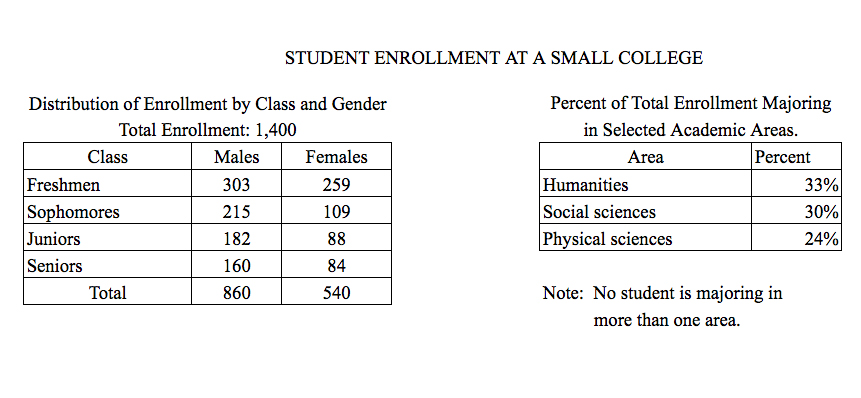# If 40 percent of the social science majors are females, how manyIf $40$ percent of the social science majors are females, how many males are social science majors?

1. $120$
2. $168$
3. $220$
4. $252$
5. $372$

So, you were trying to be a good test taker and practice for the GRE with PowerPrep online. Buuuut then you had some questions about the quant section—specifically question 15 of the second Quantitative section of Practice Test 1. Those questions testing our knowledge of Graphical Methods for Describing Data can be kind of tricky, but never fear, PrepScholar has got your back!

## Survey the Question

Let’s search the problem for clues as to what it will be testing, as this will help shift our minds to think about what type of math knowledge we’ll use to solve this question. Pay attention to any words that sound math-specific and anything special about what the numbers look like, and mark them on our paper.

Looks like we’ll need to analyze two tables containing data in this question, so it likely will draw on what we’ve learned about Graphical Methods for Describing Data. Let’s keep what we’ve learned about this skill at the tip of our minds as we approach this question.

## What Do We Know?

Let’s carefully read through the question and make a list of the things that we know.

1. $40$ percent of the social science majors are females
2. We want to know the number of social science majors who are male

## Develop a Plan

Let’s quickly scan the two tables to see what they have. The first table seems to be a two-way table for the class of a student (Freshmen, Sophomores, Juniors, or Seniors) and the gender of a student (Male or Female). The second table appears to show the percent of all students in three different majors.

We want to know how many social science majors are male. We know that $40$ percent of the social science majors are female, so it stands to reason that $100-40$, or $60$ percent of the social science majors are male.

The tables also tell us that there are $1{,}400$ total students, and that of these students $30$ percent of them are social sciences majors. Ah, looks like the solution path we’ll need to take is forming very nicely:

1. Find out the total number of social science majors by multiplying the student total by the percent who are social science majors $(30%)$
2. Find the number of male social science majors by multiplying the answer we just got for the total number of social science majors by the percent of them who are male $(60 %)$

So let’s get to it!

## Solve the Question

First, let’s calculate the total number of social science majors:

 $\Total \Social \Science \Majors$ $=$ $\Number \of \Students · \Percent \Majoring \in \Social \Science$   $\Total \Social \Science \Majors$ $=$ $1{,}400·30%$   $\Total \Social \Science \Majors$ $=$ $1{,}400·30/100$   $\Total \Social \Science \Majors$ $=$ $420$

Now let’s multiply this answer by the percent of social science majors who are male to finish answering this question.

 $\Male\Social \Science \Majors$ $=$ $\Number \of \Social \Science \Majors· \Percent \Who \are \Male$   $\Male\Social \Science \Majors$ $=$ $420·60%$   $\Male\Social \Science \Majors$ $=$ $420·60/100$   $\Male\Social \Science \Majors$ $=$ $252$

The correct answer is D, $252$.

## What Did We Learn

Here we had to calculate a nested percentage, or a percentage of a percentage. Let’s always remember that nested percentages should be solved sequentially, one right after the other. We should never try to combine them by adding or subtracting the percent change. For example, an increase of $10$ percent followed by an additional increase of $10$ percent is NOT the same as an increase of $20$ percent.

Want more expert GRE prep? Sign up for the five-day free trial of our PrepScholar GRE Online Prep Program to access your personalized study plan with 90 interactive lessons and over 1600 GRE questions.